Learning Objective

In this lesson we will learn how unstable isotopes can undergo different types of nuclear decay.

Learning Outcomes

By the end of this lesson you will be able to:

• Describe alpha, beta and gamma decay.

• Predict the products of different types of nuclear decay.

• Define half life and calculate how much of a radioactive substance will remain after a given amount of time.

• Compare the penetrating power of alpha, beta and gamma radiation.

• Define radiation dose and give examples of natural and artificial sources of radiation.

BUY THE YEAR 9 CHEMISTRY WORKBOOK

## Introduction• Most atoms are stable but a small proportion are unstable and undergo spontaneous radioactive decay.
• There are different types of radioactive decay, depending of the type of radiation emitted from the atom.
• The rate of radioactive decay and the intensity of the radiation depends on the particular isotope.
• There are natural and artificial sources of radiation, with both positive and negative effects.

## Stable and Unstable Atoms

• Most elements are made up of different isotopes, which means they can have atoms with different numbers of neutrons in their nuclei.
For example, carbon can exist as carbon-12 (with 6 protons and 6 neutrons), carbon-13 (with 6 protons and 7 neutrons) and carbon-14 (with 6 protons and 8 neutrons).
• Isotopes can be stable or unstable.
Stable isotopes are made up of atoms with balanced forces between nuclear particles (protons and neutrons).
For example, carbon-12 and carbon-13 are stable isotopes of carbon.
Unstable isotopes are made up of atoms with unbalanced forces between nuclear particles.
For example, carbon-14 is an unstable isotope of carbon.
• Most naturally occurring atoms are stable, but a small proportion are unstable.
For example, approximately 99% of carbon atoms are the carbon-12 isotope and approximately 1% of carbon atoms are the carbon-13 isotope, which means that virtually all carbon atoms are stable. However, about one in every trillion carbon atoms is the unstable carbon-14 isotope.Carbon has three naturally occurring isotopes – two are stable, one is unstable.

• Unbalanced nuclear forces in unstable isotopes are usually the result of too few or too many neutrons in the nuclei of atoms.
• As a result, unstable isotopes emit radiation, to become more stable.
Radiation is energy in the form of subatomic particles or electromagnetic waves.
• The release of radiation from the nuclei of atoms is known as radioactivity, radioactive decay or nuclear decay.
• Unstable isotopes are also known radioactive isotopes, which is usually abbreviated to radioisotopes.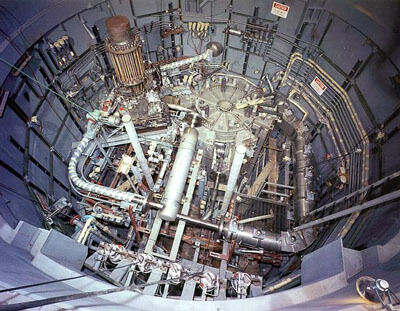The core of a nuclear reactor.

(Image: ORNL, Wikimedia Commons)

## Types of Nuclear Decay

• Nuclear decay may involve the ejection of nuclear particles, to alter the numbers of protons and neutrons, or it may just involve the release of excess nuclear energy.
• There are three main types of nuclear decay, depending on the type of radiation emitted – alpha decay, beta decay and gamma decay.
Alpha decay and beta decay involve the ejection of nuclear particles, whereas gamma decay involves the release of electromagnetic waves.
Alpha decay and beta decay result in a change in the number of protons in the nuclei of atoms and therefore a change in the type of atom – a process known as transmutation.

### Alpha Decay

• Alpha decay is the ejection of alpha particles from a nucleus.
• An alpha particle consists of two protons and two neutrons.
This is equivalent to a helium-4 nucleus, so it is often represented as.
Alpha particles can gain electrons from their environment to become neutral.
Alpha particles can also be represented using the symbol alpha, asor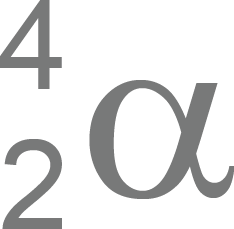.
• Alpha decay results in a decrease in atomic number and mass number.
For example, the radioisotope plutonium-239 undergoes alpha decay to form uranium -235, as shown in the following equation:Note that the sum of the atomic numbers (subscripts) and mass numbers (superscripts) is the same on both sides of the equation.
• Alpha decay occurs in large atoms with unstable nuclei.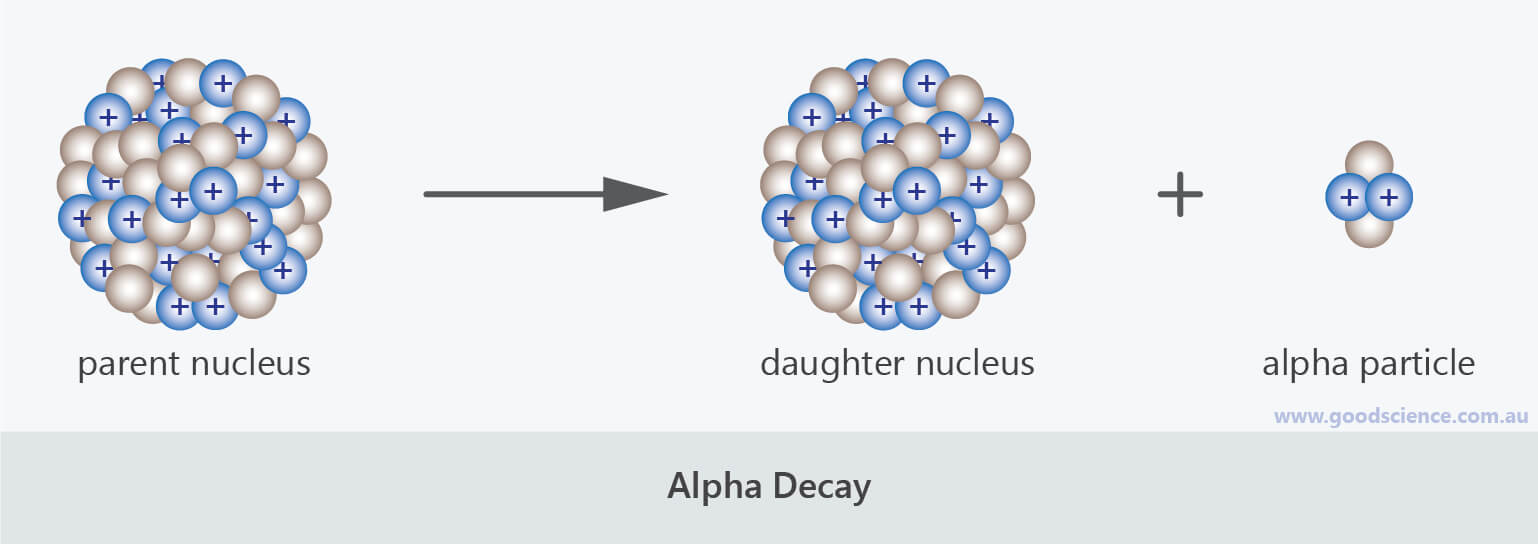Alpha decay involves the ejection of alpha particles from nuclei.

### Beta Decay

• Beta decay is the ejection of beta particles from a nucleus.
• A beta particle is the same as an electron, so it is often represented asor.
Beta particles can also be represented using the symbol beta, asor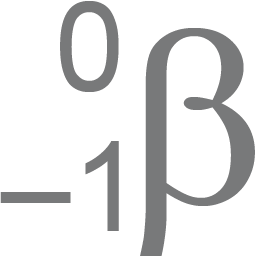.
• The beta particle ejected during beta decay is not one of the electrons orbiting the nucleus.
Instead, it forms when a neutron is converted into a proton and an electron, as shown by the following equation:Since beta particles contain no protons or neutrons, they have a mass number of 0.
They are given an atomic number of -1, so that the sum of the atomic numbers is the same on both sides of the equation.
• Beta decay results in an increase in atomic number but the mass number remains the same.
For example, the radioisotope carbon-14 undergoes beta decay to form nitrogen-14, as shown in the following equation: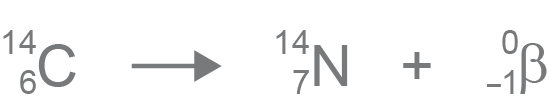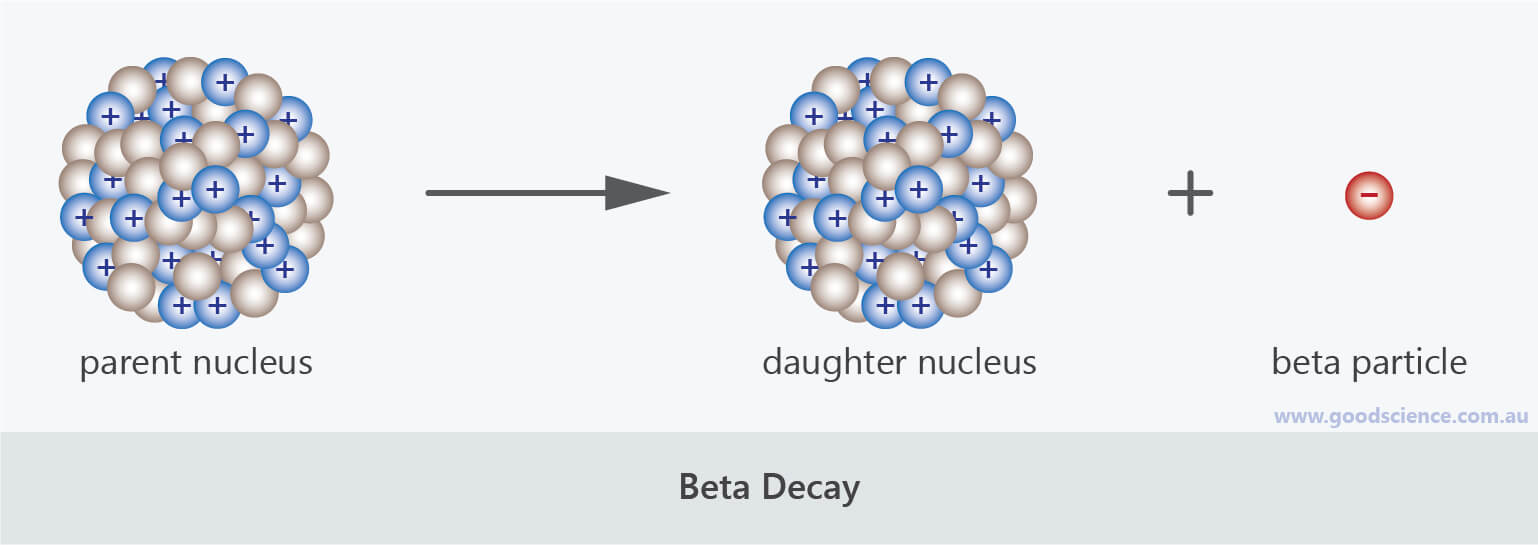Beta decay involves the ejection of beta particles from nuclei.

### Gamma Decay

• Gamma decay is the emission of gamma rays from a nucleus.
• Gamma rays are not particles, but a type of electromagnetic wave, similar to X-rays but having more energy.
• Gamma rays are represented using the symbol gamma, asor.
The latter symbol is to show that, since gamma rays are not particles, they contain no protons or neutrons and therefore their atomic number and mass number are both 0.
• Gamma radiation results from the movement of protons and neutrons in a high-energy nucleus.
• Since gamma decay does not change the number of protons or neutrons in a nucleus, the atomic number and mass number stay the same.
For example, the radioisotope cobalt-60 undergoes gamma decay as shown in the following equation:Gamma decay involves the emission of gamma rays from nuclei.

## Half Life

• The rate (speed) of nuclear decay is measured by a radioisotope’s half-life.
The half life of a radioisotope is the time it takes for half of its nuclei to undergo nuclear decay.
Depending on the isotope, this can range from a fraction of a second to billions of years.
• Example
The radioisotope sodium-24 is often used for detecting leaks in underground pipes.
It undergoes beta decay to form magnesium-24, as shown in the following equation:The half-life of sodium-24 is 15 hours.
If there were originally 100 sodium-24 atoms in a sample, after 15 hours there would be 50 sodium-24 atoms and 50 magnesium-24 atoms.
After another 15 hours there would be 25 sodium-24 atoms and 75 magnesium-24 atoms, and so on.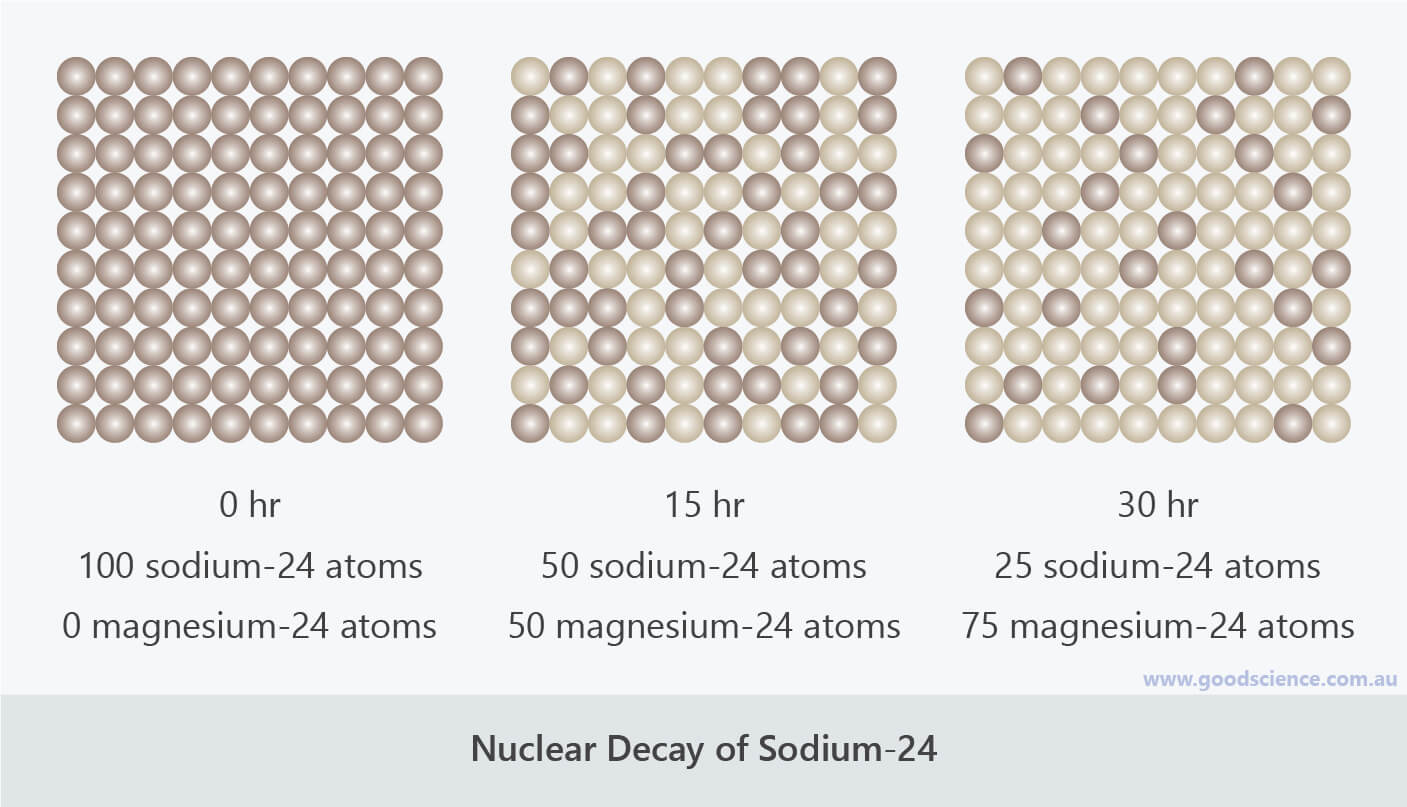Sodium-24 has a half life of 15 hours.

## Penetration and Absorption of Radiation

• As radiation moves through substances, it is absorbed by them.
As a result, the further radiation travels, the less intense it becomes.
Thicker, heavier substances are more effective at absorbing radiation.
• Each of the three types of radiation penetrate materials to different extents.
Alpha radiation is the least penetrating. It can only travel a few centimetres in air and can be stopped by a sheet of paper.
Beta radiation can travel a few metres in air and can be stopped by a sheet of aluminium foil.
Gamma radiation is the most penetrating. It can travel several hundred metres in air and requires several centimetres of lead or a thick wall of concrete to be stopped.
• All types of radiation are damaging to living things.
The more penetrating the radiation is, the more dangerous it is.
Beta and gamma radiation can cause severe burns, sickness and mutation.
Alpha radiation is relatively safely, unless inhaled or ingested – then it becomes a serious health hazard.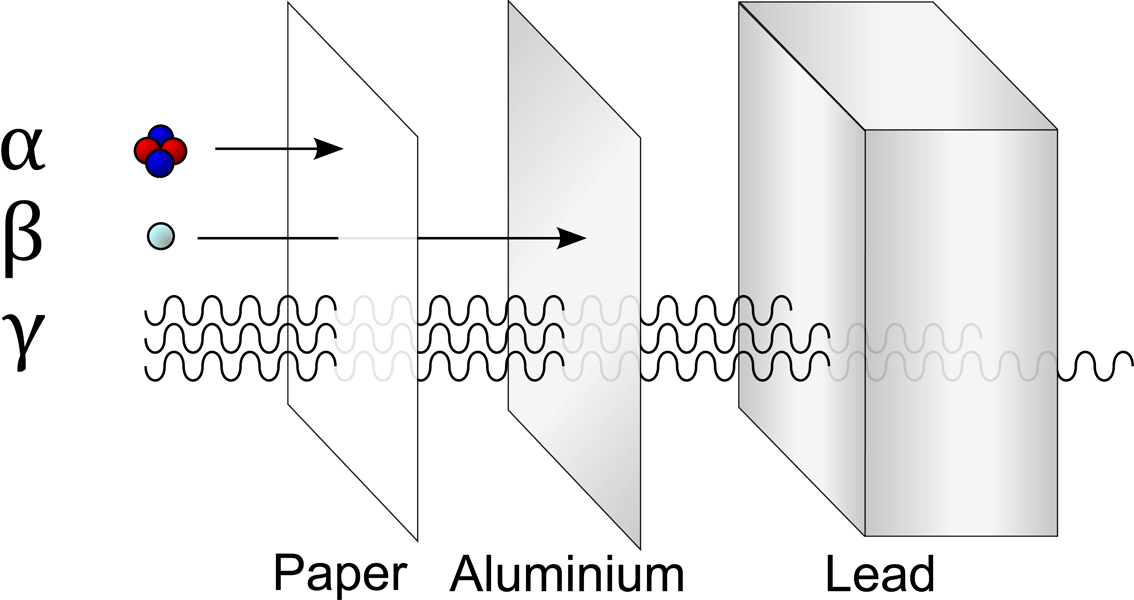Penetrating power of different types of radiation.

(Image: Ehamberg, Wikimedia Commons)

• The impact of radiation on living things depends on both the type of radiation and the amount of radiation.
• The amount of radiation absorbed by a substance is referred to as a radiation dose.
There are several different ways dose can be measured or estimated. These use the units grays (Gy) or sieverts (Sv).
• Each day we are exposed to small amounts of natural radiation, including:
• Internal radiation – radiation from within our bodies or other living things (potassium, carbon).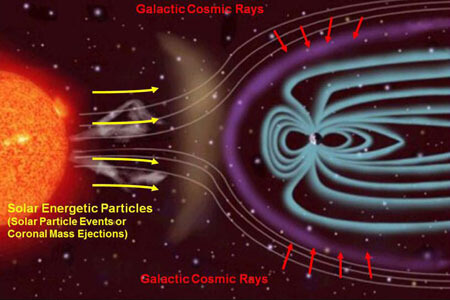(Image: NASA/JPL-Caltech/SwRI, Wikimedia Commons)

• We are also exposed to many sources of man-made radiation, including:
• Medical sources – X-rays, nuclear medicine (iodine, caesium).
• Consumer products – televisions, smoke detectors (americium), luminous watches (tritium).
• Building materials and fuels.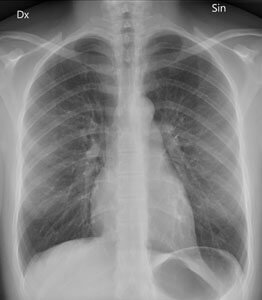(Images: jaytaix, Pixabay; Mikael Häggström, Wikimedia Commons)

• Natural sources account for the majority of radiation that the average person is exposed to.
The total dose of radiation that the average person receives, from both natural and man-made sources, has not been shown to cause harm.
However, excessive exposure to UV radiation (sunlight) or exposure to nuclear radiation (from nuclear weapons or nuclear accidents) has been shown to cause cancer.High doses of radiation can be very damaging to living things.

(Images: National Cancer Institute, Wikimedia Commons; US National Archives and Records Administration, Wikimedia Commons)

• Some professions, such as nuclear science and radiography, involve exposure to higher levels of radiation than the average person.
People who work in these industries have their radiation dose constantly measured, and there are strict limits on the amount of radiation they can be exposed to.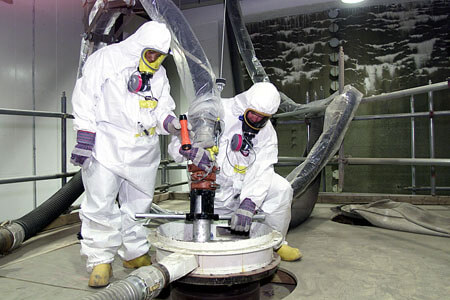People who work near radiation need to constantly monitor their radiation dose.

(Image: Fluor, Wikimedia Commons)

## Summary

• Some atoms are unstable due to unbalanced forces between nuclear particles – protons and neutrons.
• These isotopes, known as radioisotopes, undergo spontaneous nuclear decay.
• Nuclear decay involves the emission of nuclear radiation from the nuclei of radioisotopes.
• There are three main types of nuclear decay – alpha, beta and gamma.
• Alpha decay is the ejection of alpha particles (helium nuclei) from atoms.
It decreases the atomic number and therefore results in the formation of a new element (transmutation). The mass number also decreases.
• Beta decay is the ejection of beta particles (electrons) from atoms.
These electrons form when a neutron is converted into a proton and an electron.
Beta decay increases the atomic number and therefore results in the formation of a new element. The mass number remains the same.
• Gamma decay is the emission of gamma rays (electromagnetic waves) from atoms.
It does not change the atomic number (or mass number), therefore the type of element stays the same.
• The half life of a radioisotope is the time it takes for half of its nuclei to undergo nuclear decay.
• All types of radiation are damaging to living things, but each penetrates materials to different extents.
Gamma radiation is the most penetrating and alpha radiation is the least penetrating.
• Radiation dose is a measure of how much radiation is absorbed by a substance or individual.
Sources of radiation can be natural or artificial, with natural sources accounting for the majority of radiation absorbed by humans.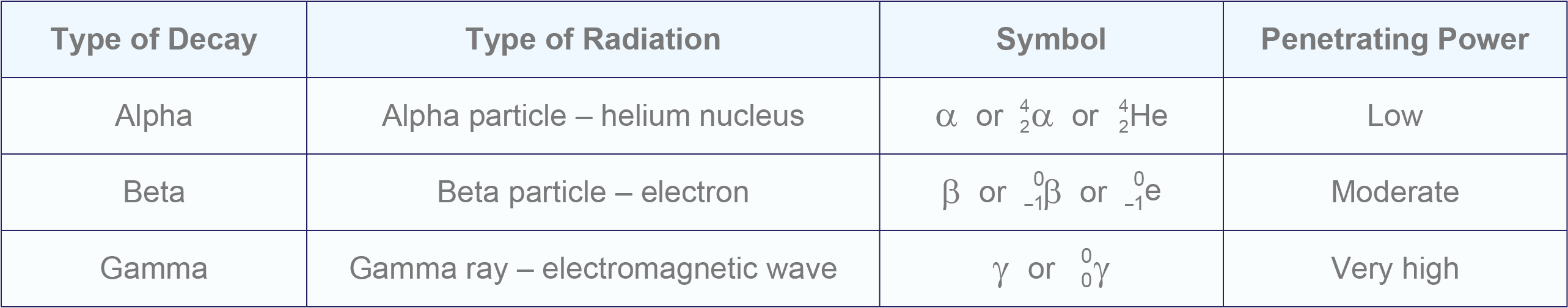Summary of the types of nuclear decay.